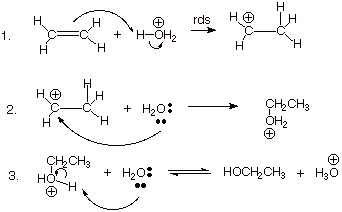# Acid Catalyzed Hydration

## Key Questions

• Acid catalyzed hydration is a chemical reaction in which water adds to an unsaturated substrate under the influence of an acid catalyst.

An example is the hydration of ethene.

CH₂=CH₂ + H-OH → H-CH₂-CH₂-OH

The common acid catalysts are sulfuric acid and phosphoric acid. They react with water to form hydronium ions, which is the strongest acid that can exist in aqueous solution.

The reaction takes place in three steps.Step 1. Protonation of the double bond.

All the hydrogen atoms in the hydronium ion are fairly positively charged because they are attached to a very electronegative oxygen atom.

One of these hydrogen atoms is strongly attracted to the carbon-carbon double bond.

The π bond breaks, and the electrons in it move to make a new bond with the hydrogen atom.

That forces the electrons in the hydrogen-oxygen bond entirely onto the oxygen.

Step 2. Formation of an oxonium ion

The carbocation reacts with one of the lone pairs on a water molecule.

A bond forms between the positively-charged carbon atom and the oxygen atom of the water. The O atom gets a positive charge

Step 3. Deprotonation of the oxonium ion

Finally, reaction with water removes one of the hydrogen atoms on the oxygen.

This regenerates the hydronium ion catalyst.

Well, you got an olefin, an electron RICH species....

#### Explanation:

i.e. $R C H = C H R$...and you could add an acid, which we will represent as ${D}_{3} {O}^{+}$...the which contains electrophilic protons/deuterons...

$R C H = C H R + \stackrel{+}{D} - O {D}_{2} \rightarrow R \stackrel{+}{C} H - C H \left(D\right) R + {D}_{2} O$

The electrophilic carbocation should then react with the hydroxyl function....

$R \stackrel{+}{C} H - C H \left(D\right) R + O {D}_{2} \rightarrow R C H \left(O D\right) - C H \left(D\right) R + {D}^{+}$

And by way of example, we could bubble ethylene gas, ${H}_{2} C = C {H}_{2}$, thru a solution containing water, and ethanol, and halide salts, and cyanide salts....there would be NO reaction UNTIL we added a source of ${H}_{3} {O}^{+}$...and this would PROTONATE the olefin to give a formal carbocation, the which would then react with ANY nucleophile present in the solvent (including the solvent itself)......

Rstackrel(+)CH-CH_2Rstackrel(H_2O,""^(-)C-=N, X^-)rarrRCH(OH)-CH_2R+RCH(C-=N)-CH_2R+RCH(X)-CH_2R